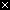# Image Code

In this section, we'll look at simple code to load and manipulate a digital image. Here we'll just manipulate one pixel at a time. In the next section, scaling up to operate on thousands of pixels at a time.

## x.png

• x.png -- tiny example image (10 x 10)
• PNG format, like JPG

The image "x.png" is very simple -- it's a very small black square with a white "x" at its center. PNG (Portable Network Graphics) is a image format, like JPG. Here is x.png:## x.png Code Example

• Three line program
• `image = new SimpleImage("x.png");` -- load into variable
• `image.setZoom(20);` -- set zoom option to 20 (10, 20, 30, ... whatever)
• `print(image);` -- print image to the right, as usual
• Zoom: each pixel shown 20x size here

Our first image code example loads the x.png image and prints it. Run the code to see what it does. Try changing the `image.setZoom(20)` to use the number 10 or 30 instead.

 image = new SimpleImage("x.png"); image.setZoom(20); print(image);

## pixel.setRed(255) Example

• `pixel = image.getPixel(0, 0);` -- upper left pixel
• `pixel.setRed(255);` -- set red value of that pixel

 image = new SimpleImage("x.png"); image.setZoom(20); pixel = image.getPixel(0, 0); pixel.setRed(255); print(image);

## Pixel Set Red/Green/Blue Functions

• `pixel.setRed(number);` -- set the red value of the pixel to be the given number (0..255)
• `pixel.setGreen(number);` -- set the green value
• `pixel.setBlue(number);` -- set the blue value

## Aside: Image Functions Reference

For later reference, there is a separate image functions reference page which lists in one place the image functions such as `pixel.setRed(number)` we are using here.

## Experiments On Pixel (0, 0)

To see how `image.getPixel(x, y)` and `pixel.setRed(number)` etc. work, we'll try the experiments listed below (use the "show" button to see the solution code).

 image = new SimpleImage("x.png"); image.setZoom(20); pixel = image.getPixel(0, 0); pixel.setRed(255); print(image);
Example ProblemsSolution
Set pixel (0, 0) to be green.
Set pixel (0, 0) to be yellow.
Set pixel (1, 0) to be yellow. Where is that pixel?
Set pixel (0, 0) to white.
Set pixel (0, 0) to be dark yellow -- set the necessary colors to 150 instead of 255.
Set pixel (0, 0) to be a light, pastel red.

< exercises